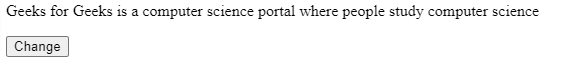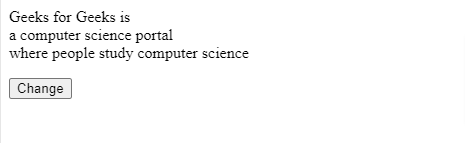# How to replace line breaks with <br> using JavaScript ?

• Last Updated : 19 Sep, 2021

Given a multiline string and the task is to replace line breaks with <br> tag.

Example:

Input: `Geeks for Geeks is
a computer science portal
where people study computer science`
Output: “Geeks for Geeks is <br> a computer science portal <br> where people study computer science”

To achieve this we have the following methods :

Method 1: Using Regex, in this example, we are creating a paragraph and a button and on clicking the button we are changing (Adding the <br>)the text of the paragraph.

We are using the String.replace() method of regex to replace the new line with <br>.

The String.replace() is an inbuilt method in JavaScript that is used to replace a part of the given string with another string or a regular expression. The original string will remain unchanged.

Example 1:

## HTML

 `` `<``html``>` `  ``<``body``>` `    ``<``p` `id``=``"para"``>`   `    `  `<``p``>` `      ``<``button` `onClick``=``"myFunc()"``>Change` `      ``<``script``>` `        ``let str = `Geeks for Geeks is ` `           ``a computer science portal ` `           ``where people study computer science`;`   `        ``let para = document.getElementById("para");`   `        ``para.innerHTML = str;`   `        ``function myFunc() {` `          ``// Replace the \n with <``br``>` `          ``str = str.replace(/(?:\r\n|\r|\n)/g, "<``br``>");`   `          ``// Update the value of paragraph` `          ``para.innerHTML = str;` `        ``}` `      ``` `    ```   `  ``` ``

Output:The initial outputAfter clicking the button

Method 2: Using split and join, in this method, we split the string from the delimiter “\n” which returns an array of substrings, and then we join the Array using the join method and pass <br /> so that every joining contains <br />.

Example 2:

## HTML

 `` `<``html``>` `  ``<``body``>` `    ``<``p` `id``=``"para"``>`   `    `  `<``p``>` `      ``<``button` `onClick``=``"myFunc()"``>Change` `      ``<``script``>` `        ``let str = `Geeks for Geeks is ` `           ``a computer science portal ` `           ``where people study computer science`;`   `        ``let para = document.getElementById("para");`   `        ``para.innerHTML = str;`   `        ``function myFunc() {` `          ``// Replace the \n with <``br``>` `          ``str = str.split("\n").join("<``br` `/>");` `          ``// Update the value of paragraph` `          ``para.innerHTML = str;` `        ``}` `      ``` `    ```   `  ``` ``

Output:The initial outputAfter clicking the button

My Personal Notes arrow_drop_up
Recommended Articles
Page :